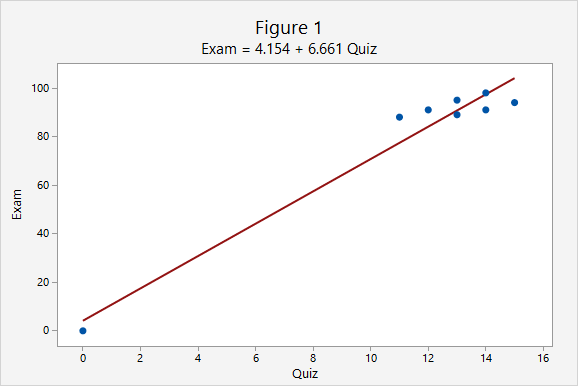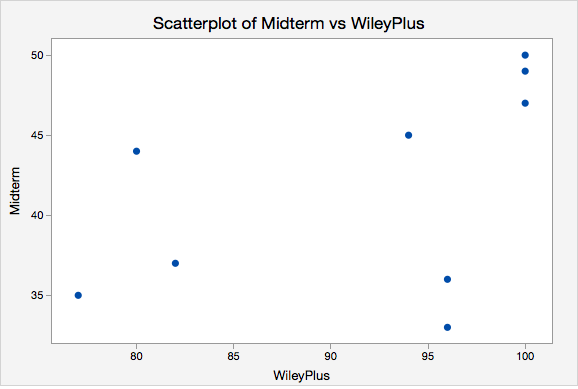# 3.4.2 - Correlation

3.4.2 - Correlation

In this course, we will be using Pearson's $r$ as a measure of the linear relationship between two quantitative variables. In a sample, we use the symbol $r$. In a population, we use the Greek letter $\rho$ ("rho"). Pearson's $r$ can easily be computed using statistical software.

Correlation
A measure of the direction and strength of the relationship between two variables.
Properties of Pearson's r
1. $-1\leq r \leq +1$
2. For a positive association, $r>0$, for a negative association $r<0$, if there is no relationship $r=0$
3. The closer $r$ is to $0$ the weaker the relationship and the closer to $+1$ or $-1$ the stronger the relationship (e.g., $r=-0.88$ is a stronger relationship than $r=+0.60$); the sign of the correlation provides direction only
4. Correlation is unit free; the $x$ and $y$ variables do NOT need to be on the same scale (e.g., it is possible to compute the correlation between height in centimeters and weight in pounds)
5. It does not matter which variable you label as $x$ and which you label as $y$. The correlation between $x$ and $y$ is equal to the correlation between $y$ and $x$.

The following table may serve as a guideline when evaluating correlation coefficients:

Absolute Value of $r$ Strength of the Relationship
0 - 0.2 Very weak
0.2 - 0.4 Weak
0.4 - 0.6 Moderate
0.6 - 0.8 Strong
0.8 - 1.0 Very strong
Cautions
1. Correlation does NOT equal causation. A strong relationship between $x$ and $y$ does not necessarily mean that $x$ causes $y$. It is possible that $y$ causes $x$, or that a confounding variable causes both $x$ and $y$.
2. Pearson's $r$ should only be used when there is a linear relationship between $x$ and $y$. A scatterplot should be constructed before computing Pearson's $r$ to confirm that the relationship is not non-linear.
3. Pearson's $r$ is not resistant to outliers. Figure 1 below provides an example of an influential outlier. Influential outliers are points in a data set that increase the correlation coefficient. In Figure 1 the correlation between $x$ and $y$ is strong ($r=0.979$). In Figure 2 below, the outlier is removed. Now, the correlation between $x$ and $y$ is lower ($r=0.576$) and the slope is less steep.Note that the scale on both the x and y axes has changed. In addition to the correlation changing, the y-intercept changed from 4.154 to 70.84 and the slope changed from 6.661 to 1.632.

# 3.4.2.1 - Formulas for Computing Pearson's r

3.4.2.1 - Formulas for Computing Pearson's r

There are a number of different versions of the formula for computing Pearson's $r$. You should get the same correlation value regardless of which formula you use. Note that you will not have to compute Pearson's $r$ by hand in this course. These formulas are presented here to help you understand what the value means. You should always be using technology to compute this value.

First, we'll look at the conceptual formula which uses $z$ scores. To use this formula we would first compute the  $z$ score for every $x$ and $y$ value. We would multiply each case's $z_x$ by their $z_y$.  If their  $x$ and  $y$ values were both above the mean then this product would be positive. If their x and y values were both below the mean this product would be positive. If one value was above the mean and the other was below the mean this product would be negative. Think of how this relates to the correlation being positive or negative. The sum of all of these products is divided by $n-1$ to obtain the correlation.

Pearson's r: Conceptual Formula

$r=\dfrac{\sum{z_x z_y}}{n-1}$
where $z_x=\dfrac{x - \overline{x}}{s_x}$ and $z_y=\dfrac{y - \overline{y}}{s_y}$

When we replace $z_x$ and $z_y$ with the $z$ score formulas and move the $n-1$ to a separate fraction we get the formula in your textbook: $r=\frac{1}{n-1}\Sigma{\left(\frac{x-\overline x}{s_x}\right) \left( \frac{y-\overline y}{s_y}\right)}$

# 3.4.2.2 - Example of Computing r by Hand (Optional)

3.4.2.2 - Example of Computing r by Hand (Optional)

Again, you will not need to compute $r$ by hand in this course. This example is meant to show you how $r$ is computed with the intention of enhancing your understanding of its meaning. In this course, you will always be using Minitab Express or StatKey to compute correlations.

In this example we have data from a random sample of $n = 9$ World Campus STAT 200 students from the Spring 2017 semester. WileyPlus scores had a maximum possible value of 100. Midterm exam scores had a maximum possible value of 50. Remember, the $x$ and $y$ variables do not need to be on the same metric to compute a correlation.

ID WileyPlus Midterm
A 82 37
B 100 47
C 96 33
D 96 36
E 80 44
F 77 35
G 100 50
H 100 49
I 94 45

Minitab Express was used to construct a scatterplot of these two variables. We need to examine the shape of the relationship before determining if Pearson's $r$ is the appropriate correlation coefficient to use. Pearson's $r$ can only be used to check for a linear relationship. For this example I am going to call WileyPlus grades the $x$ variable and midterm exam grades the $y$ variable because students completed WileyPlus assignments before the midterm exam.### Summary Statistics

From this scatterplot we can determine that the relationship may be weak, but that it is reasonable to consider a linear relationship. If we were to draw a line of best fit through this scatterplot we would draw a straight line with a slight upward slope. Now, we'll compute Pearson's $r$ using the $z$ score formula. The first step is to convert every WileyPlus score to a $z$ score and every midterm score to a $z$ score. When we constructed the scatterplot in Minitab Express we were also provided with summary statistics including the mean and standard deviation for each variable which we need to compute the $z$ scores.

Statistics
Variable N* Mean StDev Minimum Maximum
Midterm 9 41.778 6.534 33.000 50.000
WileyPlus 9 91.667 9.327 77.000 100.000
ID WileyPlus $z_x$
A 82 $\frac{82-91.667}{9.327}=-1.036$
B 100 $\frac{100-91.667}{9.327}=0.893$
C 96 $\frac{96-91.667}{9.327}=0.465$
D 96 $\frac{96-91.667}{9.327}=0.465$
E 80 $\frac{80-91.667}{9.327}=-1.251$
F 77 $\frac{77-91.667}{9.327}=-1.573$
G 100 $\frac{100-91.667}{9.327}=0.893$
H 100 $\frac{100-91.667}{9.327}=0.893$
I 94 $\frac{94-91.667}{9.327}=0.250$
z-score
$z_x=\frac{x - \overline{x}}{s_x}$

A positive value in the $z_x$ column means that the student's WileyPlus score is above the mean. Now, we'll do the same for midterm exam scores.

ID Midterm $z_y$
A 37 $\frac{37-41.778}{6.534}=-0.731$
B 47 $\frac{47-41.778}{6.534}=0.799$
C 33 $\frac{33-41.778}{6.534}=-1.343$
D 36 $\frac{36-41.778}{6.534}=-0.884$
E 44 $\frac{44-41.778}{6.534}=0.340$
F 35 $\frac{35-41.778}{6.534}=-1.037$
G 50 $\frac{50-41.778}{6.534}=1.258$
H 49 $\frac{49-41.778}{6.534}=1.105$
I 45 $\frac{45-41.778}{6.534}=0.493$

Our next step is to multiply each student's WileyPlus $z$ score with his or her midterm exam score.

ID $z_x$ $z_y$ $z_x z_y$
A -1.036 -0.731 0.758
B 0.893 0.799 0.714
C 0.465 -1.343 -0.624
D 0.465 -0.884 -0.411
E -1.251 0.340 -0.425
F -1.573 -1.037 1.631
G 0.893 1.258 1.124
H 0.893 1.105 0.988
I 0.250 0.493 0.123

A positive "cross product" (i.e., $z_x z_y$) means that the student's WileyPlus and midterm score were both either above or below the mean. A negative cross product means that they scored above the mean on one measure and below the mean on the other measure. If there is no relationship between $x$ and $y$ then there would be an even mix of positive and negative cross products; when added up these would equal around zero signifying no relationship. If there is a relationship between $x$ and $y$ then these cross products would primarily be going in the same direction. If the correlation is positive then these cross products would primarily be positive. If the correlation is negative then these cross products would primarily be negative; in other words, students with higher $x$ values would have lower $y$ values and vice versa. Let's add the cross products here and compute our $r$ statistic.

$\sum z_x z_y = 0.758+0.714-0.624-0.411-0.425+1.631+1.124+0.988+0.123=3.878$

$r=\frac{3.878}{9-1}=0.485$

There is a positive, moderately strong, relationship between WileyPlus scores and midterm exam scores in this sample.

# 3.4.2.3 - Minitab Express to Compute Pearson's r

3.4.2.3 - Minitab Express to Compute Pearson's r

## MinitabExpress – Pearson's r

We previously created a scatterplot of quiz averages and final exam scores and observed a linear relationship. Here, we will compute the correlation between these two variables.

1. Open the data set:
2. On a PC: Select Statistics > Correlation > Correlation
On a MAC: Select Statistics > Regression > Correlation
3. Double click the Quiz_Average and Final in the box on the left to insert them into the Variables box
4. Click OK

This should result in the following output:

Correlation: Quiz_Average, Final
 Pearson correlation of Quiz_Average and Final = 0.608630 P-Value = <0.0001
Video Walkthrough

Select your operating system below to see a step-by-step guide for this example.

  Link ↥ Has Tooltip/Popover Toggleable Visibility